# Pytorch入门教程11-Softmax 函数和交叉熵2020年10月10日07:50:00

1 4779字阅读15分55秒

## 关于 Softmax 和多分类问题

### Softmax 函数介绍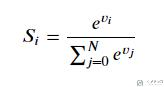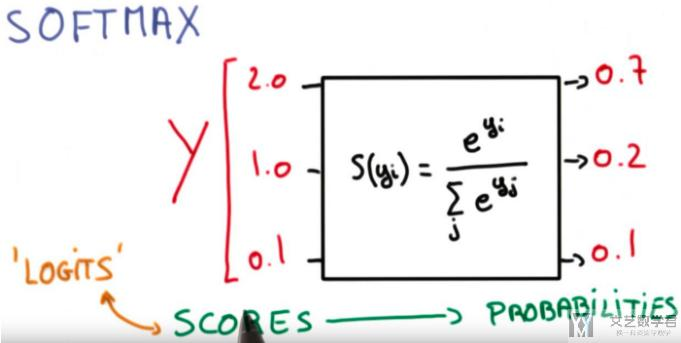1. x = torch.tensor([2, 1, 0.1])
2. s = torch.softmax(x, dim=0)
3. print('Sotfmax的输出:{}'.format(s))
4. print('Sotfmax的输出总和:{}'.format(s.sum().item()))
5. """
6. Sotfmax的输出:tensor([0.6590, 0.2424, 0.0986])
7. Sotfmax的输出总和:1.0000001192092896
8. """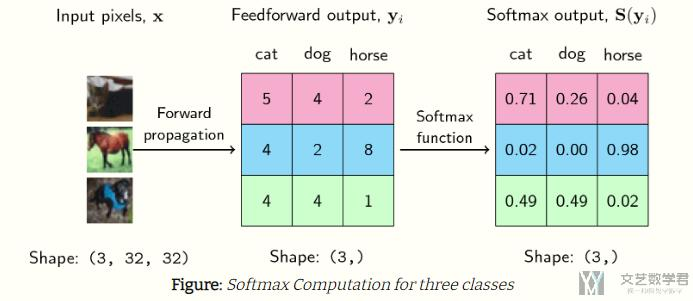The output of the softmax describes the probability (or if you may, the confidence) of the neural network that a particular sample belongs to a certain class.

Thus, for the first example above, the neural network assigns a confidence of 0.71 that it is a cat, 0.26 that it is a dog, and 0.04 that it is a horse. The same goes for each of the samples above.

### 交叉熵损失函数• 对于两个模型的准确率来说，是一样的，准确率都是 `2/3`
• 对于「模型一」来说，他在`数据 1``数据 2` 上，对结果的确定性都不是很确定，都是 `0.4`，只比 `0.3` 大一点；
• 对于「模型二」来说，他的预测结果就表现很肯定，对结果的可能性都是 `0.7`，会远远比其他类别要大。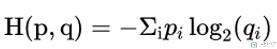• p(真实的分布)，在实际中也就是 0 和 1，是正确的 label 就是 1，否则就是 0；
• q(预测的概率)，模型给出的概率（经过 softmax），都是介于 0-1 之间的数字；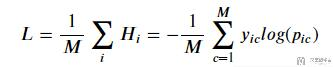• `M` 为总的数据的数量；
• `y` 是实际标签, 为 `one-hot` 编码；
• `p` 为模型输出的概率；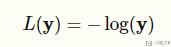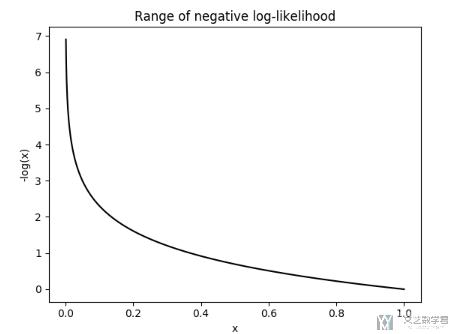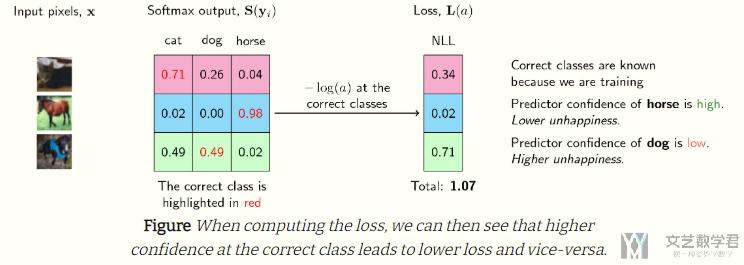1. loss = nn.NLLLoss()
2. Y = torch.tensor([2, 1, 0])
3. # 模型1对每条数据的预测，每条数据对应三个概率，表示该条数据属于第 i 类的概率值
4. model_one_pred = torch.tensor(
5.     [[0.3, 0.3, 0.4],  # predict class 2
6.      [0.3, 0.4, 0.3],  # predict class 1
7.      [0.1, 0.2, 0.7]])  # predict class 2
8. # 模型2对每条数据的预测，每条数据对应三个概率，表示该条数据属于第 i 类的概率值
9. model_two_pred = torch.tensor(
10.     [[0.1, 0.2, 0.7],  # predict class 2
11.      [0.1, 0.7, 0.2],  # predict class 1
12.      [0.4, 0.3, 0.3]])  # predict class 2l1 = loss(Y_pred_good, Y)
13. l1 = loss(torch.log(model_one_pred), Y)
14. l2 = loss(torch.log(model_two_pred), Y)
15. l1, l2
16. # > (tensor(1.3784), tensor(0.5432))

## 关于 Sotfmax 和 NLLLoss 的数学推导

### 数学推导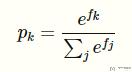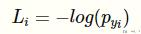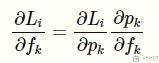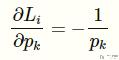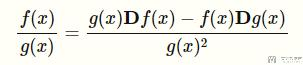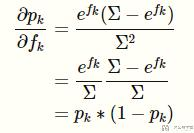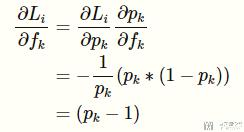1. loss = nn.CrossEntropyLoss(reduction='sum')
2. # 实际的分类
3. Y = torch.tensor([0, 2, 1])
4. # 模型一对每条数据的预测，每条数据对应三个概率，表示该条数据属于第 i 类的概率值
5. X = torch.tensor(
6.     [[5, 4, 2],  # predict class 2
7.      [4, 2, 8],  # predict class 1
8.      [4, 4, 1]], dtype=torch.float32, requires_grad=True)  # predict class 2
9. l = loss(X, Y)
10. print('loss:{}'.format(l.item()))
11. # >> loss:1.0873291492462158

1. p = nn.Softmax(dim=1)
2. print(p(X))
3. """
4. tensor([[0.7054, 0.2595, 0.0351],
5.         [0.0179, 0.0024, 0.9796],
7. """

1. -torch.log(p(X))
2. """
3. tensor([[0.3490, 1.3490, 3.3490],
4.         [4.0206, 6.0206, 0.0206],
6. """

1. l.backward()  # 反向传播，计算梯度
3. """
4. X的梯度,
5. tensor([[-0.2946,  0.2595,  0.0351],
6.         [ 0.0179,  0.0024, -0.0204],
7.         [ 0.4879, -0.5121,  0.0243]])
8. """

1. p(X)-1
2. """
3. tensor([[-0.2946, -0.7405, -0.9649],
4.         [-0.9821, -0.9976, -0.0204],
6. """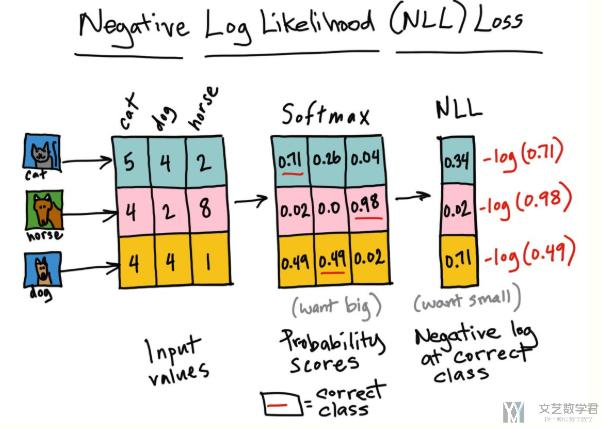• 微信公众号
• 关注微信公众号
•• QQ群
• 我们的QQ群号
•• 本文由 发表于 2020年10月10日07:50:00
• 转载请务必保留本文链接：https://mathpretty.com/12538.html